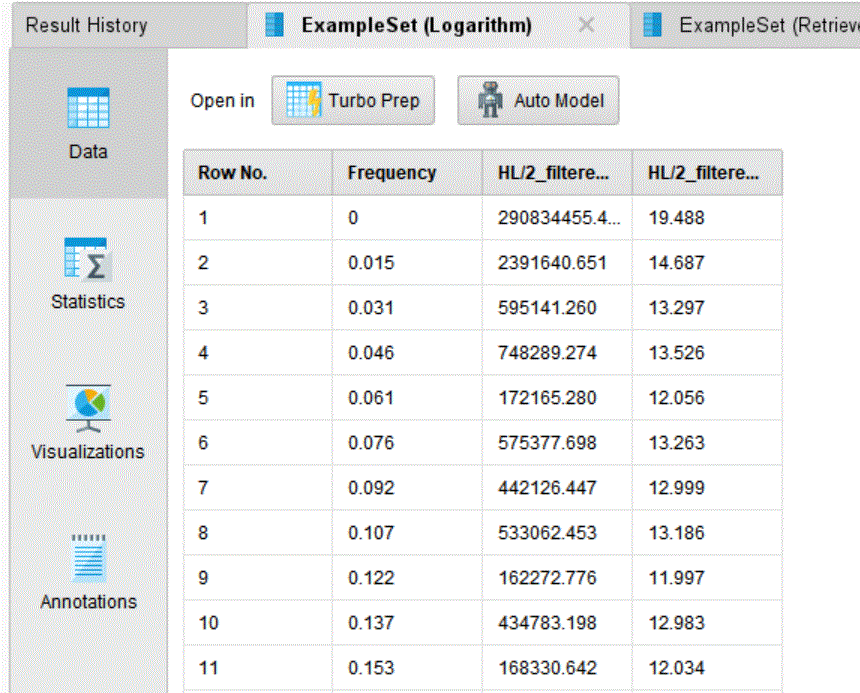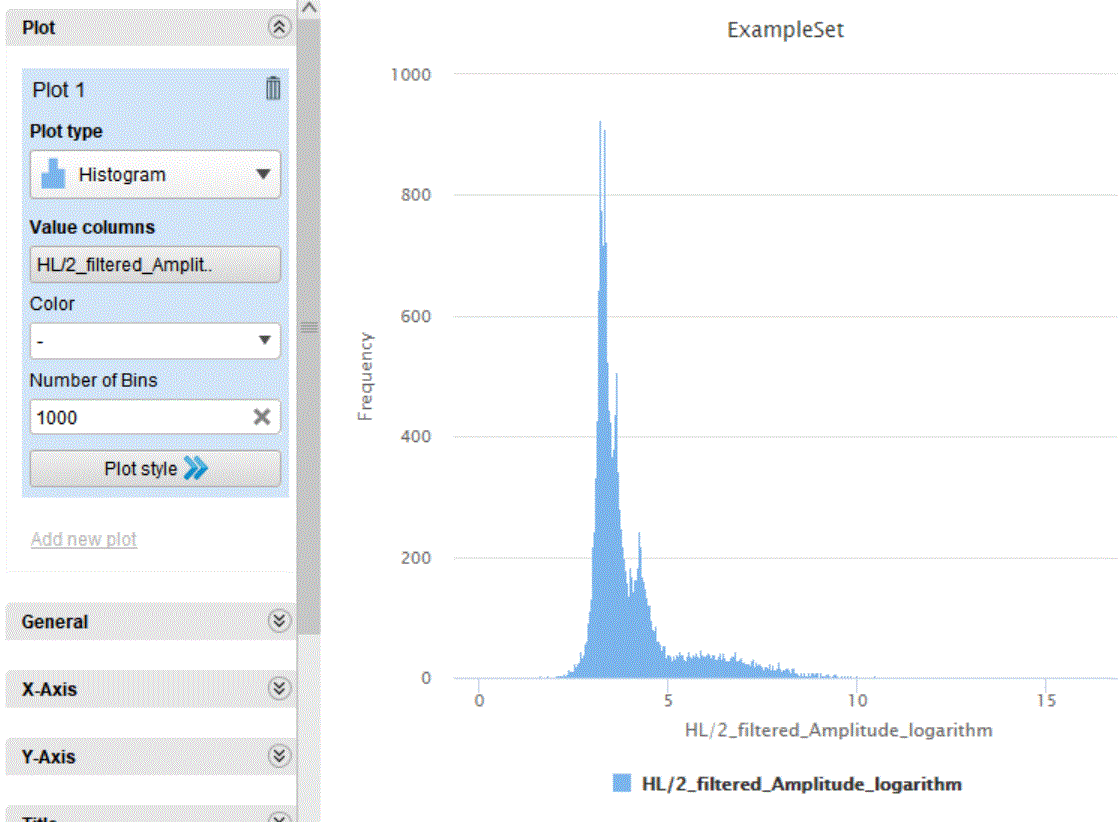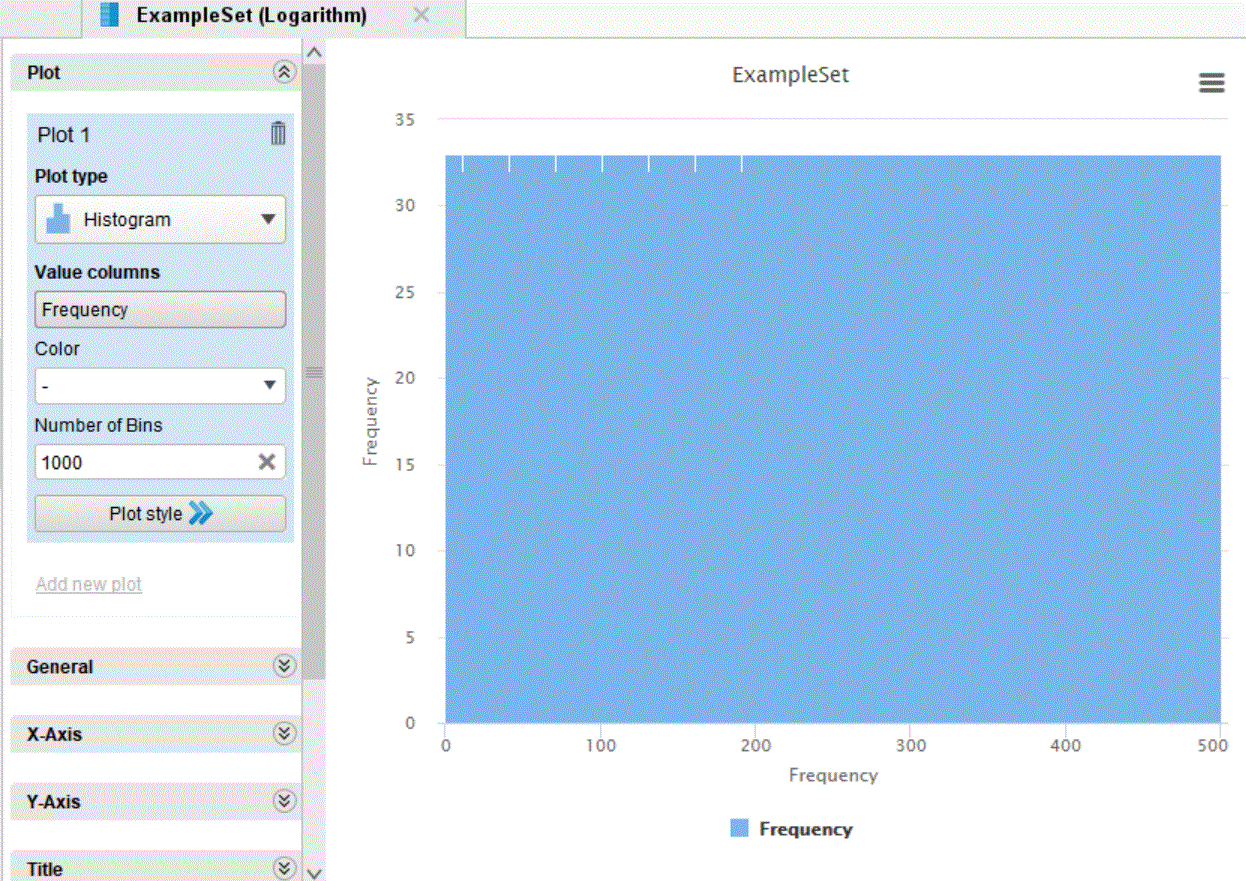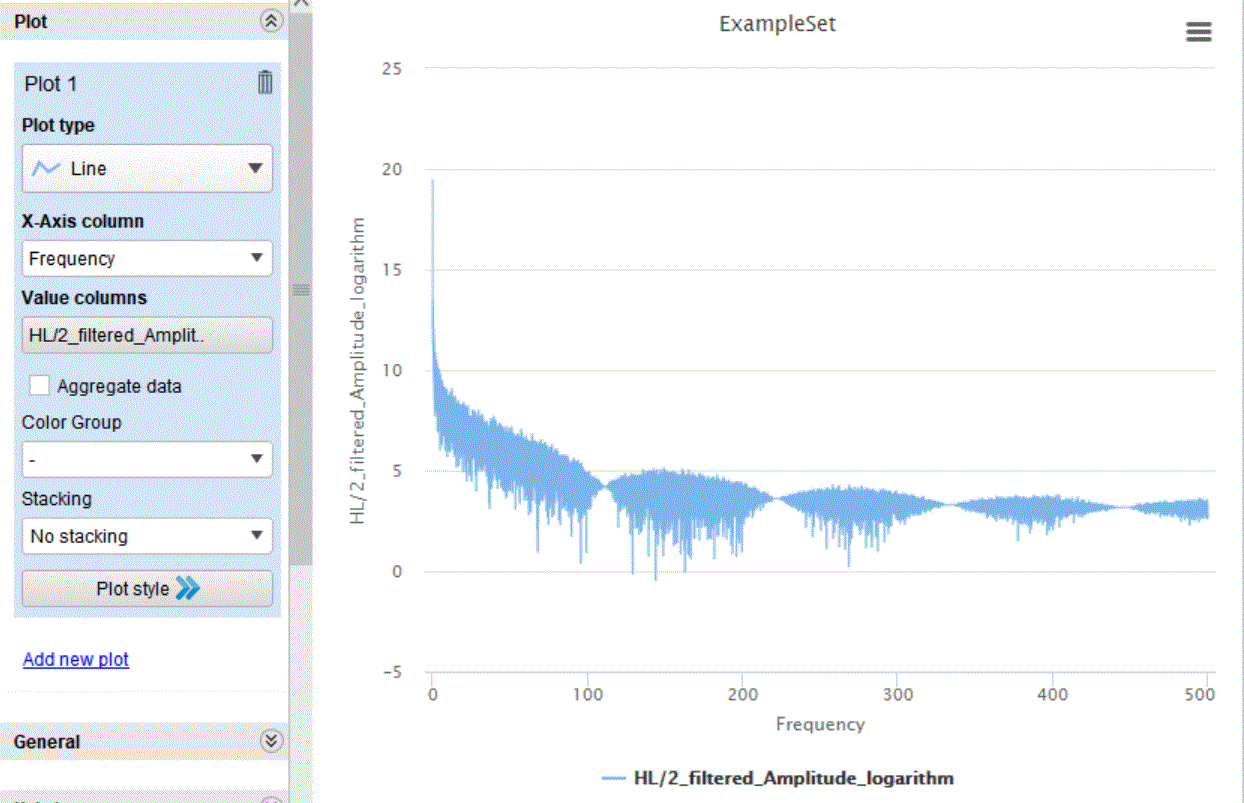# HELP: FFT CHART OF A FINANCIAL FUTURE MOVING AVERAGE

Member Posts: 1Newbie
Dear All,

I am a terrific newbie on rapidminer. I need to extract dominant cycles (peaks of frequencies) on a time series of the financial tools, in the example of S&P future, candlesticks on 1-minute timeframe. I have previously calculated per minute the average price value (high+low)/2 and then the moving average on 10 periods (minutes) to smooth a bit of noise. Then I have calculated the FFT. The calculation is made generating frequency but not considering the timestamp column since there are ranges of time during which there are no exchanges (Saturday, Monday, night...) and they have not to count az missing or zero values. Said this, I have at this point several questions. The generated FFT is as in the following screenshot table, on the FFT amplitudes it has been applied a log since some components have too high magnitude than othersif I display the FFT spectrum as a Histogram I obtain the following result:The shape could be coherent, but not the labels on the X and Y axes that appear inverted. I have selected as values columns "HL/2_filtered_amplitude" and what I expect is that this field is the Y axe. So the first question is

Q1: why axes are inverted?

and

Q2: despite the labels, is the histogram really showing on the Y the amplitude of the frequency spectrum and on X the frequency?

Note that if I change the values columns field and select the "frequency" column I get a flat histogram.Q3: how can I pot correctly the FFT amplitude with an histogram chart?

If I change the type of chart for instance lines and set on Y and X the proper columns I get something very different and with an unexpected shapeQ4: is this chart showing correctly the X and Y axes?

The other big question is on frequency interpretation: provided that

1. i do not declare the timestamp column
2. the values are given with a time interval of 1 minute
3. i do not know the number of samples considered
4. I checked the "calculate frequency" option

Q5: What does the number on the frequency axe represent? is it correct to interpret the N value as a period 1/N minutes?